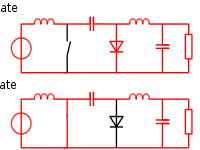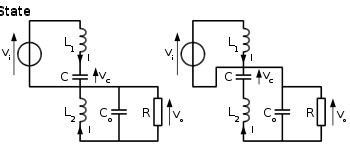# Ćuk converter

﻿
Ćuk converter

The Ćuk converter (pronounced Chook, sometimes incorrectly spelled Cuk, Čuk or Cúk) is a type of DC-DC converter that has an output voltage magnitude that is either greater than or less than the input voltage magnitude.

The non-isolated Ćuk converter can only have opposite polarity between input and output. It uses a capacitor as its main energy-storage component, unlike most other types of converters which use an inductor. It is named after Slobodan Ćuk of the California Institute of Technology, who first presented the design.

## Non-isolated Ćuk converter

### Operating PrincipleFig 1: Schematic of a non-isolated Ćuk converter.Fig 2: The two operating states of a non-isolated Ćuk converter.Fig 3: The two operating states of a non-isolated Ćuk converter. In this figure, the diode and the switch are either replaced by a short circuit when they are on or by an open circuit when they are off. It can be seen that when in the Off state, the capacitor C is being charged by the input source through the inductor L1. When in the On state, the capacitor C transfers the energy to the output capacitor through the inductance L2.

A non-isolated Ćuk converter comprises two inductors, two capacitors, a switch (usually a transistor), and a diode. Its schematic can be seen in figure 1. It is an inverting converter, so the output voltage is negative with respect to the input voltage.

The capacitor C is used to transfer energy and is connected alternately to the input and to the output of the converter via the commutation of the transistor and the diode (see figures 2 and 3).

The two inductors L1 and L2 are used to convert respectively the input voltage source (Vi) and the output voltage source (Co) into current sources. Indeed, at a short time scale an inductor can be considered as a current source as it maintains a constant current. This conversion is necessary because if the capacitor were connected directly to the voltage source, the current would be limited only by (parasitic) resistance, resulting in high energy loss. Charging a capacitor with a current source (the inductor) prevents resistive current limiting and its associated energy loss.

As with other converters (buck converter, boost converter, buck-boost converter) the Ćuk converter can either operate in continuous or discontinuous current mode. However, unlike these converters, it can also operate in discontinuous voltage mode (i.e., the voltage across the capacitor drops to zero during the commutation cycle).

### Continuous mode

In steady state, the energy stored in the inductors has to remain the same at the beginning and at the end of a commutation cycle. The energy in an inductor is given by:$E=\frac{1}{2}LI^2$

This implies that the current through the inductors has to be the same at the beginning and the end of the commutation cycle. As the evolution of the current through an inductor is related to the voltage across it:$V_L=L\frac{dI}{dt}$

it can be seen that the average value of the inductor voltages over a commutation period have to be zero to satisfy the steady-state requirements.

If we consider that the capacitors C and Co are large enough for the voltage ripple across them to be negligible, the inductor voltages become:

• in the off-state, inductor L1 is connected in series with Vi and C (see figure 2). Therefore VL1 = ViVC. As the diode D is forward biased (we consider zero voltage drop), L2 is directly connected to the output capacitor. Therefore VL2 = Vo
• in the on-state, inductor L1 is directly connected to the input source. Therefore VL1 = Vi. Inductor L2 is connected in series with C and the output capacitor, so VL2 = Vo + VC

The converter operates in on-state from t=0 to t=D·T (D is the duty cycle), and in off state from D·T to T (that is, during a period equal to (1-D)·T). The average values of VL1 and VL2 are therefore:$\bar V_{L1}=D \cdot V_i +\left(1-D\right)\cdot\left(V_i-V_C\right) =\left(V_i-(1-D)\cdot V_C\right)$$\bar V_{L2}=D\left(V_o+V_C\right) + \left(1-D\right)\cdot -V_o=\left(V_o + D\cdot V_C\right)$

As both average voltage have to be zero to satisfy the steady-state conditions we can write, using the last equation:$V_C=\frac{V_o}{D}$

So the average voltage across L1 becomes:$\bar V_{L1}=\left(V_i+(1-D)\cdot \frac{V_o}{D}\right)=0$

Which can be written as:$\frac{V_o}{V_i}=\frac{D}{1-D}$

It can be seen that this relation is the same as that obtained for the Buck-boost converter.

### Discontinuous mode

Like all DC-DC converters Cuk converters rely on the ability of the inductors in the circuit to provide continuous current, in much the same way a capacitor in a rectifier filter provides continuous voltage. If this inductor is too small or below the "critical inductance", then the current will be discontinuous. This state of operation is usually not studied too much depth, as it is not used beyond a demonstrating of why the minimum inductance is crucial.

The minimum inductance is given by:$L_1min=\frac{(1-D)^2R}{2Df_s}$

Where fs is the switching frequency.

## Related structures

### Inductor coupling

Instead of using two discrete inductor components, many designers implement a coupled inductor Ćuk converter, using a single magnetic component that includes both inductors on the same core. The transformer action between the inductors inside that component gives a coupled inductor Ćuk converter lower output ripple than a Ćuk converter using two independent discrete inductor components.

### Single-ended primary-inductance converter (SEPIC)

A SEPIC converter is able to step-up or step-down the voltage.

Wikimedia Foundation. 2010.

### Look at other dictionaries:

• CUK — may refer to:* CUK is the ICAO airline designator for Polo Aviation, United Kingdom * CUK is the IATA airport code for Caye Caulker Airport, Belizeee also*Ćuk converter used in electronics *Çük an Idel Ural festival …   Wikipedia

• Ćuk-Wandler — Als Ćuk Wandler (englisch Ćuk converter) bezeichnet man in der Elektronik eine diskrete elektronische Schaltung, die eine elektrische Gleichspannung in eine andere elektrische Gleichspannung transformieren kann. Der Betrag der… …   Deutsch Wikipedia

• Convertidor Ćuk — El convertidor Ćuk es un tipo de convertidor DC DC en el cual la magnitud de voltaje en su salida puede ser inferior o superior a su voltaje de entrada. El convertidor Ćuk no aislado solo puede tener polaridad opuesta entre su entrada y su salida …   Wikipedia Español

• DC-to-DC converter — An assortment of 78xx series ICs, linear DC DC converters A DC to DC converter is an electronic circuit which converts a source of direct current (DC) from one voltage level to another. It is a class of power converter. Contents …   Wikipedia

• Convertisseur Cuk — Convertisseur Ćuk Un convertisseur Ćuk est une alimentation à découpage qui convertit une tension continue en une autre tension continue de plus faible ou plus grande valeur mais de polarité inverse. Au contraire des autres types de… …   Wikipédia en Français

• Convertisseur Ćuk — Un convertisseur Ćuk est une alimentation à découpage qui convertit une tension continue en une autre tension continue de plus faible ou plus grande valeur mais de polarité inverse. Au contraire des autres types de convertisseurs, qui utilisent… …   Wikipédia en Français

• Commutation cell — The commutation cell is the basic structure in power electronics. It is composed of an electronic switch (today a high power semiconductor, not a mechanical switch) and a diode. It was traditionally referred to as a chopper, but since switching… …   Wikipedia

• Switched-mode power supply — switched mode power supply. A bridge rectifier B Input filter capacitors C Transformer D output filter coil E output filter capacitors ] A switched mode power supply, switching mode power supply or SMPS, is an electronic power supply unit (PSU)… …   Wikipedia

• Gleichspannungswandler — Ein Gleichspannungswandler, auch DC DC Wandler genannt, englisch DC DC Converter, bezeichnet eine elektrische Schaltung, welche eine am Eingang zugeführte Gleichspannung in eine Gleichspannung mit höherem, niedrigerem oder invertiertem… …   Deutsch Wikipedia

• Convertisseur SEPIC — Figure 1: Schéma de base d un convertisseur SEPIC Un convertisseur SEPIC de l acronyme de (single ended primary inductor converter) est une alimentation à découpage convertissant une tension continue en une autre tension continue, de valeur… …   Wikipédia en Français